# Mom and daughter

Mom is 30 years older than the daughter. What is the age difference between them in 35 years?

x =  30

### Step-by-step explanation:

$x=\left(30+35+y\right)-\left(35+y\right)=30+35+y-35-y=30$We will be pleased if You send us any improvements to this math problem. Thank you!Tips to related online calculators
Do you have a linear equation or system of equations and looking for its solution? Or do you have a quadratic equation?

## Related math problems and questions:

• Mom and daughterMother is 39 years old. Her daughter is 15 years. For many years will mother be four times older than the daughter?
• The familyHow old is a mother if she is four times older than her daughter and 5 years ago she was even seven times older than her daughter? Today, a son is 30 years younger than a father. 7 years ago, a father was seven times older than a son. How old is a son tod
• Father and daughterFather is 36 years old, daughter is 20 years less. What will be the ratio between them when they are 10 years more?
• Family and age ratiosThe age of father and son are in the ratio 10:3; the age of father and daughter are in the ratio 5:2. How old are father and son if the daughter is 20 years old? In what ratio is age sister and brother?
• Three siblings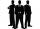Three siblings have birthday in one day-today. Together they have 35 years today. The youngest is three years younger than middle and the oldest is 5 years older than middle. How old is each?
• The father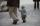The father is 36 years old. The son is 6 years old. How many years will a father be 3 times older than a son?
• Television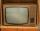When we add to the difference between the numbers 1704 and 654 the difference numbers 14920 and 14042, we get a year in which television was invented. How many years people known the TV?
• TroopsThere are two leaders in the tourist group, with an average age of 30 years and several children with an average age of 10 years. The total age of the group is 12 years. How many children are in the group?
• Numbers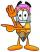By how many is the difference of numbers 8 and 34 less than its product?
• The average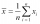The average of one set of 4 numbers is 35. The average of another set of number is 20. The average of the numbers in the two sets is 30. How many numbers are there in the other set?
• Mystery numberA mystery number is between 800 and 920. No digits are the same. The difference of the numbers 5. What is the mystery number?
• Multiples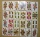What is the sum of the multiples of number 7 that are greater than 30 but less than 56?
• Father and son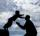Father is 44 years old, his son 16 years. Determine how many years ago was the father five times older than the son.
• Mother and daughter 2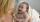The mother is 40 years older than her daughter. How old is the mother if her age is eight thirds age of daughter?
• Perctentages35% of what number is 35?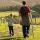Daddy is a 46 year old and son is at age of 16. When (which year) was/will daddy 5 times more years than his son?The mother is four times older than her daughter. Five years ago, her daughter was seven times younger than her mother. How many years do they have now?